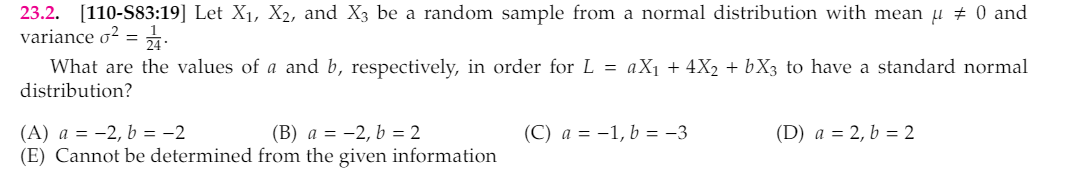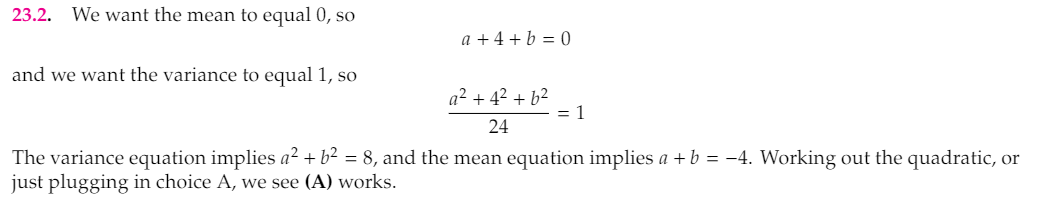# Question about the variance of normal distribution

Home Forums SOA Exams Question about the variance of normal distribution

Viewing 1 post (of 1 total)
• Author
Posts
• #4961I am confused with the equation a+4+b=0 and the following variance equation. In my opinion, in order to let the combined mean to be zero, it should be:

a * (mean of X1) + 4 * (mean of X2) + b * (mean of X3) = 0

and for the variance part, it should be:

(a^2) * (variance of X1) + (4^2) * (variance of X2) + (b^2) * (variance of X3) = 1

Appreciate for the help.

Viewing 1 post (of 1 total)
• You must be logged in to reply to this topic.